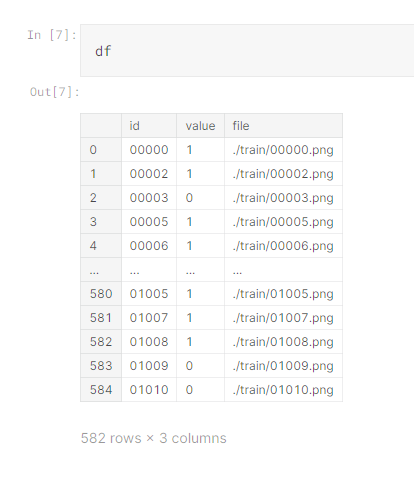# How does FastAI implicitly convert a vector to a number when outputing a prediction?

Hi, could someone please help me figure out what the default behavior is here? Docs aren’t very specifc:

I have a net like this:

``````import torch
import torch.nn as nn
import torch.nn.functional as F

class Net(nn.Module):
def __init__(self, pretrained=False):
super().__init__()
self.conv1 = nn.Conv2d(3, 6, 5)
self.pool = nn.MaxPool2d(2, 2)
self.conv2 = nn.Conv2d(6, 16, 5)
self.fc1 = nn.Linear(16 * 5 * 5, 120)
self.fc2 = nn.Linear(120, 84)
self.fc3 = nn.Linear(84, 10)

def forward(self, x):
x = self.pool(F.relu(self.conv1(x)))
x = self.pool(F.relu(self.conv2(x)))
x = torch.flatten(x, 1) # flatten all dimensions except batch
x = F.relu(self.fc1(x))
x = F.relu(self.fc2(x))
x = F.relu(self.fc3(x))
return x
``````

I create a learner like this:

``````learn = cnn_learner(dls, Net, metrics=[error_rate, accuracy], model_dir="/tmp/model/").to_fp16()
``````

So my net technically returns a vector of length 10, but the dls I have has training data labelled 0 or 1. AFAIK the default loss function is CrossEntropyLoss, but when making predictions using `learn.predict()`, how does FastAI convert the vector to a single number? It definitely manages to do it somehow as I am able to get back predictions after training, but would like specifics on what it’s doing, is it like a softmax?

Hi Daniel

If you have only 2 labels then the 10 below should be 2. if it was 2 it would return the probability of of label 0 and label 1. So say you had cats or dogs it would return 0.9 cat and 0.1 dog.
`self.fc3 = nn.Linear(84, 10)`

Regards Conwyn

Thanks for the reply Conwyn, my question is more so how does my original code above actually manage to work? Is there some implicit one-hot representation it converts my labels to to a 10-vector so that it can do the regression?

I think probably what happens, is that FastAI converts the labels to a one-hot representation like this,

0 → [1,0,0,0,0,0,0,0,0,0]
1 → [0,1,0,0,0,0,0,0,0,0]

so the last 8 spots of the label vector (ground truth) are just never used. This is the only way I see it working. Can anyone confirm?

I believe then `predict` must be just taking the values in the first two spots of the output vector as the predictions, even though there would be 10 spots.

``````dls = ImageDataLoaders.from_df(df, item_tfms=Resize(224), bs=64, label_col =1, fn_col=2, path='')